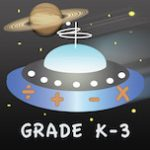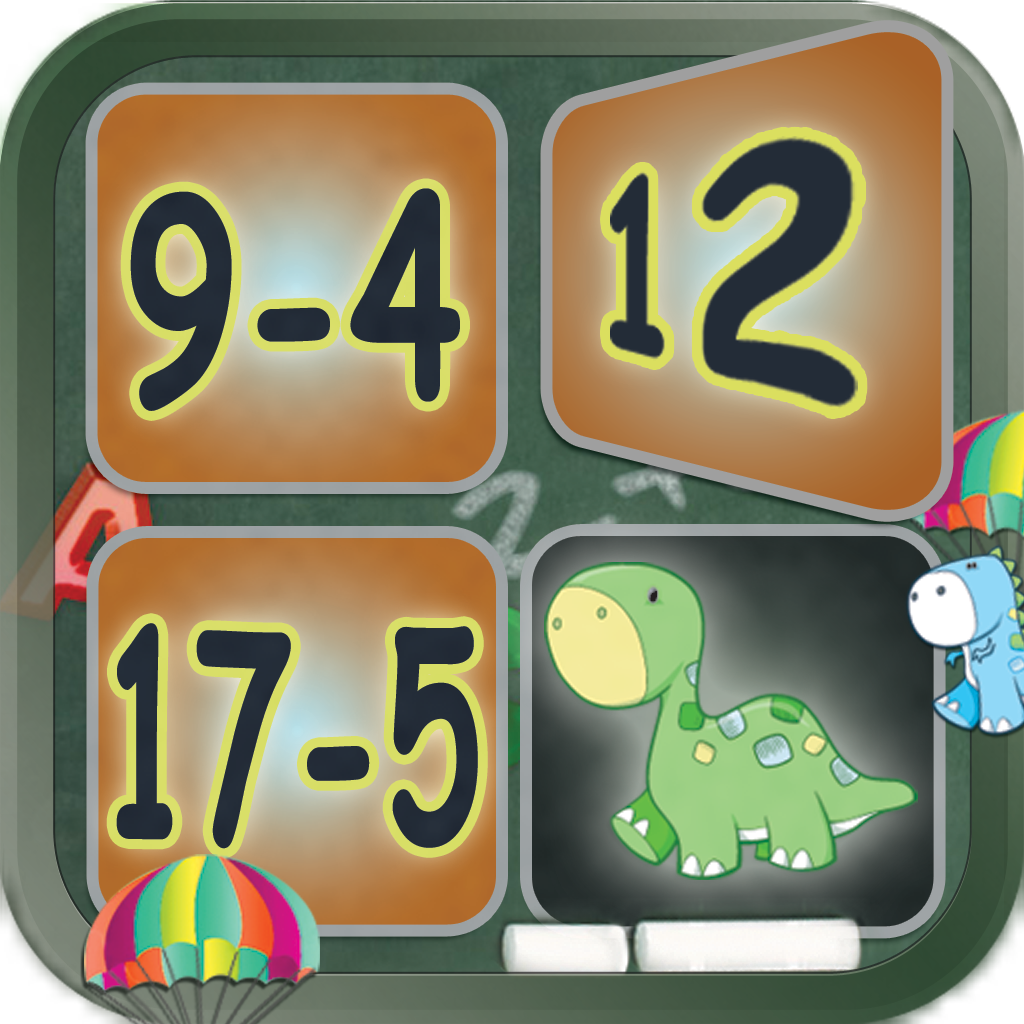## 2nd Grade Printable Math Quiz WorksheetsYou can download a number of math practice worksheets designed for Grade 2  Elementary School. The practice worksheets are based on Math Essentials textbook and math common core standards. Unit 1 covers the following concepts: add and subtract numbers up to 20 add three or four numbers find partners and totals write equations for math mountains tell […]

## 5th Grade Printable Math Quiz SheetsThis page offers a number of math worksheets for Grade 5 based on Math Essentials math textbook and common core standards. Unit 1 covers the following topics: – fractions – simplify fractions – addition and subtraction of fractions – word problems with fractions Tap/click the following links to print math tests from Unit 1: 1. 5th Grade Unit 1 […]This page offers a number of math worksheets for Grade 4 following Math Essentials math textbook and common core standards. The worksheets are organized in four different lessons (Units). The Math topics in the first lesson (Unit) are as follows: – Calculate area and perimeter of triangle, square, rectangle, parallelogram, or trapezoid – Order of […]The app is a reliable follow-up resource for common core standardized lessons. Astro Math: Grades K-3 by @Reks includes math facts covering kindergarten through 3rd grade school curriculum. By protecting each planet individually a student goes through levels practicing grade-level appropriate math skills (counting, addition, subtraction, multiplication, division). The student collects coins upon successfully completed missions and […]

## Multiplication: Math Facts Card Matching...This educational memory game is a great tool for building a good foundation for math that today’s school curriculum requires.  A student becomes a faster player with every new game using Multiplication: Math Facts Card Matching Game by @Reks. The application also represents a classic matching game traditionally used to build cognitive skills.

## Subtraction: Math Facts Card Matching Ga...Students of all ages and abilities can benefit using this application.  Subtraction: Math Facts Card Matching Game is designed to encourage higher level thinking and reasoning in a fun, and friendly atmosphere. * great tool for helping children subtract a number accurately and quickly * three modes: single-player mode(practice); two-player mode (play with a friend); […]